# Solution assignment 09 Optimizing f(x)

### Assignment 9

Verify whether the function: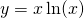has a maximum or a minimum.

### Solution

We calculate the derivative (product rule) and make it equal to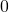: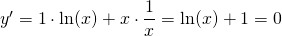This equation has the solution: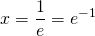In order to verify whether we have a maximum or a minimum, we calculate the second derivative:For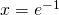there is a minimum with the coordinates:0